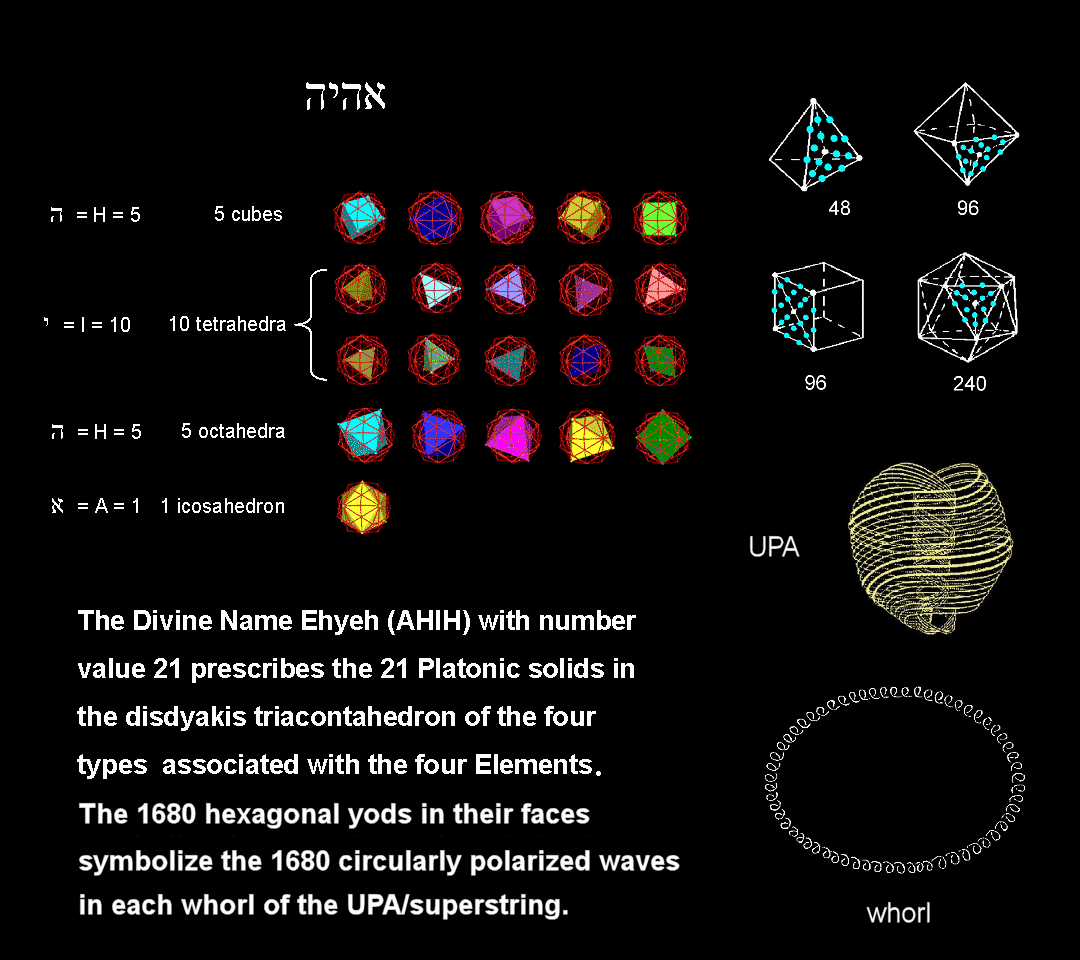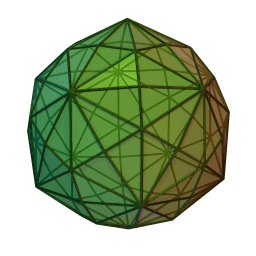<< Previous    1...   3  4    6  7  ...54    Next >>

#5 The faces of the 21 Platonic solids of the first four types in the disdyakis triacontahedron have 1680 hexagonal yodsDisdyakis triacontahedron

The numbers of hexagonal yods in the faces of the tetrahedron, octahedron, cube & icosahedron when their sectors are tetractyses are, respectively, 48, 96, 96 & 240. Ten tetrahedra, five octahedra, five cubes & one icosahedron, i.e., 21 Platonic solids, can be fitted in the disdyakis triacontahedron. These numbers are the letter values of EHYEH (AHIH), the Godname with number value 21 of Kether, which is the first Sephirah of the Tree of Life (see here). The number of hexagonal yods in their faces is 1680:

10 tetrahedra: 10×48 = 480
5 octahedra: 5×96 = 480
5 cubes: 5×96 = 480
1 icosahedron: 1×240 = 240

Total = 1680

They symbolize the 1680 circular turns in each helical whorl of the UPA, the subquark state of the E8×E8 heterotic superstring, as remote-viewed by Annie Besant & C.W. Leadbeater (see here). The ancient Greeks believed that these Platonic solids were the shapes of the particles of the Elements Earth, Water, Air & Fire. As the polyhedral version of the outer Tree of Life, the disdyakis triacontahedron "contains" these solids in such numbers that their faces require 1680 hexagonal yods when constructed from tetractyses. This is another way in which this polyhedron determines the oscillatory form of the whorl in the basic particle making up the atomic nuclei of all the elements. The reason for this is that the disdyakis triacontahedron is the polyhedral counterpart of the universal blueprint embodied in various sacred geometries. Hence, its geometry "contains" the primary number that quantifies the way in which this blueprint is realized in the subatomic world. Every holistic system is parameterized by a unique set of numbers, and the number 1680 is just one of these, although it is a primary parameter. It is one of the ways in which the number obtained paranormally by C.W. Leadbeater through his remote-viewing superstrings is embodied in the disdyakis triacontahedron.

 << Previous    1...   3  4    6  7  ...54    Next >>

Home